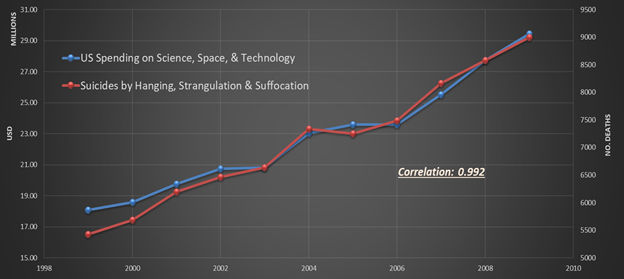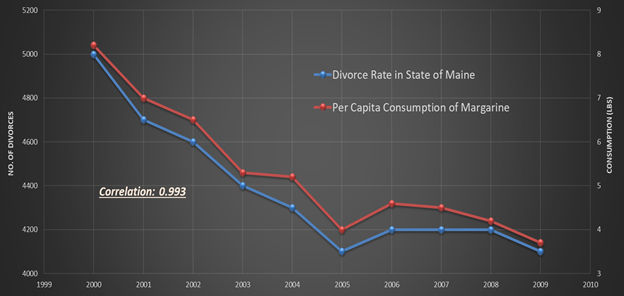# The Main Characteristics of Engineering Application of Artificial Intelligence (PART ONE)Shahab Mohaghegh

2 years ago | 6 min read

The Main Characteristics of Engineering Application of Artificial Intelligence (PART ONE)

Outline
Introduction — (Part One)
Explaining Traditional Engineering Models — (Part One)
Explaining Models of Physics Developed by AI & Machine Learning (XAI) — (Part Two)
- Part 1 of XAI: Key Performance Indicators (KPI) — (Part Two)
- Part 2 of XAI: Sensitivity Analysis — (Part Two)
— Step One: Single Parameter Sensitivity Analysis — (Part Two)
— Step Two: Double Parameters Sensitivity Analysis — (Part Two)
— Step Three: Multiple Parameters Sensitivity Analysis — (Part Two)
- Part 3 of XAI: Type Curves — (Part Three)
— Explainable AI Model for Unconventional Reservoirs (Shale Analytics) — (Part Three)
— Explainable AI Model for Conventional Reservoirs (Top-Down Modeling) — (Part Three)
History of Explainable AI (XAI) in the Petroleum Data Analytics — (Part Four)
Conclusions — (Part Four)

Introduction

Engineering Application of Data Science can be defined as using Artificial Intelligence and Machine Learning to model physical phenomena purely based on facts (field measurements, data). The main objective of this technology is the complete avoidance of assumptions, simplifications, preconceived notions, and biases.

One of the major characteristics of Engineering Application of Data Science is its incorporation of Explainable Artificial Intelligence (XAI).

While using actual field measurements as the main building blocks of modeling physical phenomena, Engineering Application of Data Science incorporates several types of Machine Learning Algorithms including artificial neural networks, fuzzy set theory, and evolutionary computing.

Predictive models of Engineering Application of Data Science (data-driven predictive models) are not represented through unexplainable “Black Box”. Predictive models of Engineering Application of Data Science are reasonably explainable.

In early 1990s, when Artificial Intelligence & Machine Learning started to be used to solve engineering related problems, engineers and scientists started asking how this technology achieves its predictive objectives.

What recently is being addressed as Explainable AI (XAI) for the non-engineering application of AI and Machine Learning, is not new in the context of engineering application of this technology.

The main reason behind the fact that engineering application of AI and Machine learning to a large extend is quite explainable has to do with the historical problems of the application of traditional statistics in solving engineering related problems.

While traditional statistics’ main solutions are about identification of “correlations” in the data, engineers and scientists were always interested in “causations” that are capable of explaining “correlations”.

This has always been one of the main problems associated with the use of traditional statistics to solve problems. There are still many data-driven solutions that are referred to as “Black Box”. Engineering application of AI and Machine Learning does not generate “Black Box” solutions.

Questions by engineers and scientists gave rise to research and development efforts in early 2000 and resulted in what today is called Explainable AI (XAI). In this article history of what today is call Explainable AI (XAI) is shown through 7 technical papers in the oil and gas industry that were published between 2001 to 2010.

Historically, prior to the development of Artificial Intelligence and Machine Learning, traditional statistics was used to analyze data. The major role of traditional statistics is to specify hypotheses that can fit the collected data.

Therefore, the key behind the traditional statistics is “correlation” while engineers and scientists have always been interested in “causation”. It is a well-established fact that “correlation” does not necessarily determine and/or represent “causation”.

Figure 1 and Figure 2 are good examples of how “correlation” does not have anything to do with “causation”. These figures demonstrate how data collected for different variables can be correlated to each other while absolutely having nothing to do with one another.

The objective of this article is to cover and illustrate Explainable AI (XAI) and demonstrate its initiation as part of Petroleum Data Analytics almost two decades ago. Different sections of this article will cover how models that are developed through Petroleum Data Analytics can explain the physics of the models that are generated using purely data-driven AI-based algorithms that do not use any data generated by mathematical equations.Figure 1. In ten years, from 1999 to 2009, U.S. spending on Science, Space and Technology highly correlates with Suicide by Hanging, Strangulations, and Suffocation. Such correlation has absolutely nothing to do with causation.Figure 2. In nine years, from 2000 to 2009, Divorce Rate in the State of Maine highly correlates with Per Capita consumption of Margarine. It is quite clear that such correlation has absolutely nothing to do with causation.

It is a well-known fact that models of physical phenomena that are generated through mathematical equations can be explained. This is one of the main reasons behind the expectation of engineers and scientists that any potential model of the physical phenomena should be explainable.

Explainability of the traditional models of the physical phenomena is achieved through the solutions of the mathematical equations that are used to build the models. Explanation of such models are achieved through the analytical (for reasonably simple mathematical equations) or numerical (for complex mathematical equations) solutions of the mathematical equations.

Solutions of the mathematical equations provide the opportunities to get answers to almost any questions that might be asked from the model of the physical phenomena.

Solutions of the mathematical equations are used to explain why and how certain results are generated by the model. It allows examination and explanation of the influence and impact of all the involved parameters (variables) on each other and on the models results (output parameters).

In general, this is the definition of engineering approach to problem solving. Therefore, results of the physical phenomena that are based on mathematical equations (no matter how simple or complex) can be explained in detail.

Figure 3 shows the Fetkovich “Type Curve”. Including early time (driving from transient flow equations) and late time curves (boundary dominated flow, Arp’s Decline Curves). Following are (Equation 1 and Equation 2) the equations that are used for this series of “Type Curves”:

Dimensionless rate (qD) and time (tD) are used in Equation 1 and Equation 2 are defined as shown below (Equation 3 and Equation 4) in the context of well-testing domain:

It can be clearly seen that the curves in Figure 3 are very “well-behaved” (continuous, non-linear, certain shape that changes in a similar fashion from curve to curve). The reason behind the “well-behaved” characteristics of these curves is the mathematical equations that were used to generate them.

All “Type Curves” that have been historically generated in the petroleum industry are very “well-behaved” because they all are developed using the solutions of the mathematical equations. Figure 4 demonstrates two more examples of such “well-behaved” “Type Curves”.

This means that traditional, mathematics-based, models of the physical phenomena are “explainable”. Physical phenomena that are developed using mathematical equations are “explainable” through performing several types of analyses of the mathematical equations.

Here are four ways of explaining physical phenomena models that are developed and solved using mathematical equations:

1. Identification of the influence of each parameter (variable) in the mathematical equation, also known as Key Performance Indicators (KPI),

2. Single-Parameter Sensitivity Analysis,

3. Multiple-Parameters Sensitivity Analysis, and

4. “Type Curve” Generation.

Performance of such analyses can provide the required explanation of any question that might be asked regarding why, and how certain outcomes are generated or certain forecasts and predictions are made based on the solutions of the mathematical equations that have been used to model the physical phenomena.

Figure 3 and Figure 4 demonstrate examples of “Type Curves” that are generated through the solutions of certain mathematical equations.

UpvoteCreated by

Shahab Mohaghegh

A pioneer in the application of Artificial Intelligence and Machine Learning in the Exploration and Production industry, director of WVU-LEADS (Laboratory for Engineering Application of Data Science). Including more than 30 years of research and development in the petroleum engineering application of AI&ML, has authored three books (Shale Analytics – Data Driven Reservoir Modeling – Application of Data-Driven Analytics for the Geological Storage of CO2), more than 200 technical papers, 60 projects. SPE Distinguished Lecturer and Distinguished Author in SPE’s Journal of Petroleum Technology. Founder of SPE’s Technical Section dedicated to AI&ML. Honored by the U.S. Secretary of Energy for AI-based technical contribution in the aftermath of the Deepwater Horizon and member of U.S. Secretary of Energy’s Technical Advisory Committee on Unconventional Resources in two represented USA in the International Standard Organization (ISO) on Carbon Capture and Storage technical committee 2014-16Post

Upvote

Downvote

Comment

Bookmark

Share

Related Articles